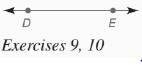Chapter 7.CR, Problem 10CR### Elementary Geometry for College St...

6th Edition
Daniel C. Alexander + 1 other
ISBN: 9781285195698

#### Solutions

Chapter
Section### Elementary Geometry for College St...

6th Edition
Daniel C. Alexander + 1 other
ISBN: 9781285195698
Textbook Problem
6 views

# In Review Exercises 7 to 13, sketch and describe the locus in a plane.Find the locus of points that are 1 2 in. from D E ↔ .To determine

To find: The locus of points that are 12 inch from DE.

Explanation

Given:

The line with points D and E is shown below

Definition used:

A locus is the set of all points and only those points that satisfy a given condition (or set of conditions).

Calculation:

Consider the given figure.

The points that are 12 inch from DE are the two parallel lines in either side of DE.

That is, each line that are 12 inch from DE and the opposite of it

### Still sussing out bartleby?

Check out a sample textbook solution.

See a sample solution

#### The Solution to Your Study Problems

Bartleby provides explanations to thousands of textbook problems written by our experts, many with advanced degrees!

Get Started

#### Evaluate the expression sin Exercises 116. 3(2)0

Finite Mathematics and Applied Calculus (MindTap Course List)

#### True or False: converges mean exists.

Study Guide for Stewart's Multivariable Calculus, 8th

#### ex(ex)2 = a) ex3 b) e3x c) 3ex d) e4x

Study Guide for Stewart's Single Variable Calculus: Early Transcendentals, 8th